• 多项logistic回归在医学统计中广泛应用，但对于大多数医学生来说是一难点，分享我找到的相关资料
• 本文脉络:logistic回归模型的含义logistic模型的决策边界函数分析logistic模型的参数最优化logistic回归模型与感知机模型的比较总结logistic回归模型的含义我们把分类模型分成两个阶段，推断阶段和决策...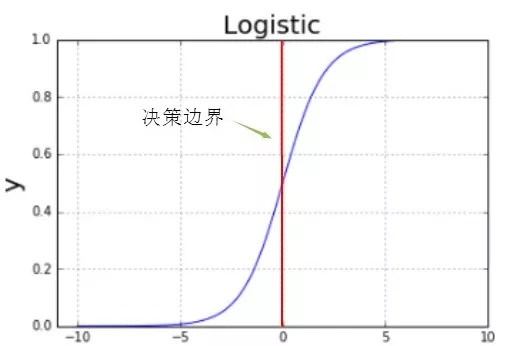logistic回归模型是一种线性生成模型。本文将介绍logistic回归模型相关的知识，为了更好地理解模型的决策边界函数，本文同时分析了多元变量的协方差对概率分布的影响。
本文脉络:
logistic回归模型的含义logistic模型的决策边界函数分析logistic模型的参数最优化logistic回归模型与感知机模型的比较总结
logistic回归模型的含义
我们把分类模型分成两个阶段，推断阶段和决策阶段，推断阶段对联合概率分布建模，然后归一化，得到后验概率。决策阶段确定每个新输入x的类别。
我们用推断阶段的方法来推导logistic回归模型，首先对类条件概率密度和类先验概率分布建模，然后通过贝叶斯定理计算后验概率密度。

考虑二分类的情形，类别C1的后验概率密度;令则:式中的就是logistic函数

因此，logistic回归的值等于输入变量为x的条件下类别为C1的概率(1) 当分类结果为C1

(2) 当分类结果为C2

结论：logistic回归值表示所属类的后验概率，无论是二分类还是多分类，分类结果都是后验概率最大所对应的类。
logistic的决策边界函数分析
决策边界函数，简而言之，就是函数的两侧是不同的分类结果。
可定性的分析协方差的三种情况与分布图的关系。
(a) 图表示正常的协方差矩阵的高斯分布图。(b) 图表示协方差矩阵是对角矩阵的高斯分布图。(c) 图表示协方差矩阵是对角矩阵且对角元素都相等的高斯分布图。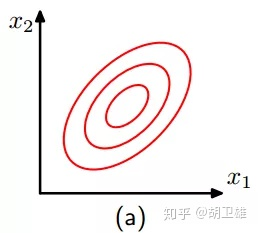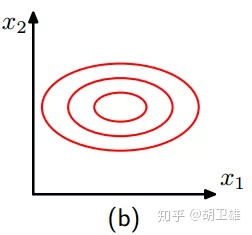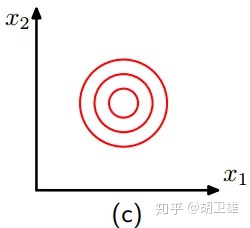logistic的决策边界函数分析
logistic曲线如下图，红色直线(a=0)表示决策边界函数: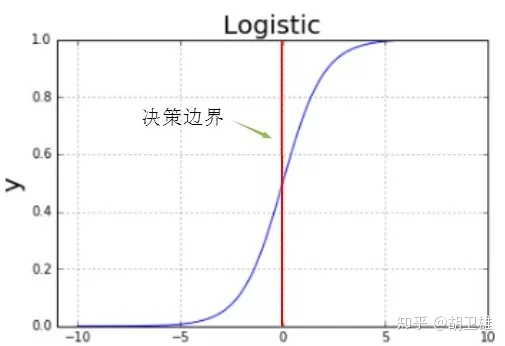假设类条件概率密度是高斯分布，即P(x|Ck),然后求解后验概率的表达式，即P(Ck|x)。我们知道，logistic回归值就是所求的后验概率。
假设类条件概率密度的协方差相同，类条件概率密度为:由上面的推导公式得后验概率为:其中:由后验概率的表达式可知，当类条件的协方差矩阵相等时，决策边界函数是随x线性变化的直线。

结论：如下图，若两类的条件概率密度的协方差相同时（如C1和C2的协方差相同），则决策边界函数是直线；若两类的条件概率密度的协方差不相同时（如C1和C3，C2和C3），则决策边界函数是曲线。判断协方差矩阵是否相同可以根据分布图形形状是否相同来判断，如C1和C2的协方差相同，C3和C1、C2的协方差不相同。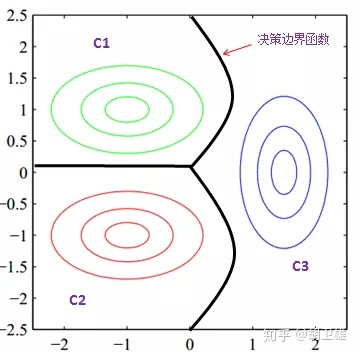假设类条件概率密度符合高斯分布且具有相同的协方差矩阵，则决策边界函数是一条直线；若类条件概率密度符合更一般的指数分布且缩放参数s相同，决策边界函数仍然是一条直线。
logistic模型的参数最优化
logistic模型损失函数
logistic回归模型的含义是后验概率分布，因此可以从概率的角度去设计损失函数。
考虑两分类情况，假设有N个训练样本，logistic模型是表示后验概率y=1的概率，则表示y=0的概率，变量取值1或0，且分别代表模型因此，似然函数损失函数logistic模型的参数最优化
损失函数最小化等价于模型参数的最优化，如下图:利用梯度下降法求最优解，学习速率:具体求法本文不介绍，只给出算法的思想。为了避免过拟合问题，则在原来的损失函数增加正则项，然后利用梯度下降法求最优解，这里也不展开。
logistic模型与感知机模型的比较
logistic模型与感知机模型的相同点
由上面的分析可知，假设类条件概率分布的协方差相同，则logistic模型的决策边界函数是随x线性变化的直线，因此，感知机模型与logistic模型的分类策略一样，即决策边界函数是一样的。如下图: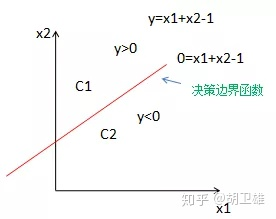感知机模型：当点落在直线上方，y>0,则分类结果为C1；反之为C2。logistic模型：当点落在上方，y>0，则后验概率P(C1|X)>0.5,分类结果为C1；反之为C2。
考虑到对输入变量x进行非线性变换,感知机和logistic模型的分类策略仍一样，决策边界函数相同，如下图: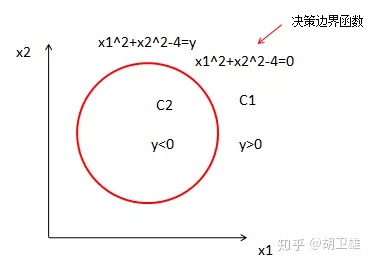感知机模型:当点落在圆外，y>0，则分类结果为C1；反之为C2。logistic模型：当点落在圆外，y>0，则后验概率P(C1|X)>0.5,分类结果为C1；反之为C2。
logistic模型与感知机模型的异同点
(1) logistic回归模型限制值的范围在0~1,感知机模型对值范围没有限制，因此logistic模型相比感知机模型，对异常点有更强的鲁棒性。如下图，当有异常数据时，logistic模型要好于感知机模型。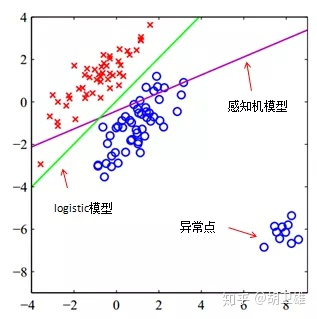(2) 感知机模型用误分类点到超平面的距离衡量损失函数，而logistic模型则从概率角度去衡量损失函数。
总结
logistic回归的含义是后验概率分布，用概率的角度去设计似然函数，logistic模型相比于感知机模型对异常数据具有更好的鲁棒性。

展开全文• 结合实际情况，可以将Logistic回归分析分为3类，分别是二元Logistic回归分析、多元有序Logistic回归分析和多元无序Logistic回归分析，如下图。Logistic回归分析用于研究X对Y的影响，并且对X的数据类型没有要求，X...在研究X对于Y的影响时，如果Y为定量数据，那么使用多元线性回归分析(SPSSAU通用方法里面的线性回归)；如果Y为定类数据，那么使用Logistic回归分析。
结合实际情况，可以将Logistic回归分析分为3类，分别是二元Logistic回归分析、多元有序Logistic回归分析和多元无序Logistic回归分析，如下图。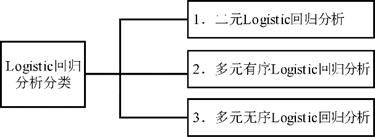Logistic回归分析用于研究X对Y的影响，并且对X的数据类型没有要求，X可以为定类数据，也可以为定量数据，但要求Y必须为定类数据，并且根据Y的选项数，使用相应的数据分析方法。
本次内容将针对二元logistic(logit)回归进行说明，后续两篇文章将分别讲解有序logistic(logit)和多分类logistic(logit)回归。
1、二元logistic分析思路说明
在进行二元Logistic回归分析时，通常会涉及3个步骤，分别是数据处理、卡方分析和影响关系研究，如下图。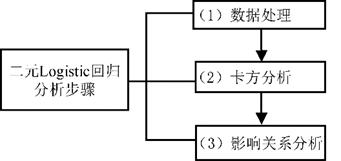1.1 第一步为数据处理
例如，在研究相关因素对样本将来是否愿意购买理财产品的影响情况时，性别，专业等均为影响因素，而且明显的，性别和专业属于定类数据，因此需要进行虚拟哑变量设置，可使用【数据处理->生成变量】完成。
除此之外，二元logistic回归要求因变量只能为2项，而且数字一定是0和1，数字1表示YES，愿意，购买，患病等，数字0表示no，不愿意，不购买，不患病等。如果不是这样，那么就需要针对因变量Y进行数据编码，使用【数据处理->数据编码】即可完成。
1.2 第二步为卡方分析或方差分析
此步不是必需的步骤，通过此步可以试探性了解每个影响因素X与Y之间的影响关系情况，研究影响关系前，首先需要自变量X与Y之间有着差异关系，才可能进一步有着影响关系，也或者说差异关系是一种基础性关系，影响关系是更进一步的深层次关系。所以在进行二元logistic回归分析前，可先对X做差异分析，筛选出与Y有着差异性的X。
如果X是定类数据，那么就使用卡方分析去分析差异；如果说X是定量数据，那么可使用方差分析去研究X和Y的差异性。
分析完成X与Y的差异关系之后，筛选出有差异的X，然后再放入模型中，进行二元logistic回归；这样做的目的有两个，一是简化模型，越简单的模型越容易拟合且效果越好；二是做到心里有数，提前了解到数据之间的大致关系情况。
1.3 第三步为影响关系分析，即二元Logistic回归分析
在上一步确认了可能的影响因素之后，此步骤直接对题进行二元Logistic回归分析。二元Logistic回归分析时，首先需要看某个题是否呈现出显著性(如果P值小于0.05，则说明呈现出0.05水平的显著性；如果P值小于0.01，则说明呈现出0.01水平的显著性)，如果呈现出显著性，那么说明该题对Y有影响关系。具体是正向影响还是负向影响需要结合对应的回归系数值进行说明，如果回归系数值大于0，则说明是正向影响；反之则说明是负向影响。
除此之外，二元Logistic回归分析会涉及一个术语——对数比(SPSSAU中称其为OR值)。对数比是一个倍数概念指标，该值等于回归系数的指数次方，也称exp(b)值。例如，研究相关因素对样本‘是否购买理财产品’的影响，性别因素呈现出显著性，并且性别以女性为对照项，其对数比(OR值)为1.34，这说明男性样本购买理财产品的可能性是女性样本的1.34倍。
二元Logistic回归分析模型的拟合情况或模型效果的判断会涉及3个指标，分别是Hosmer和Lemeshow检验、R2值和模型预测准确率表格。Hosmer和Lemeshow检验用于检验事实数据情况与模型拟合结果是否保持一致，如果在进行Hosmer和Lemeshow检验时P值大于0.05，那么说明事实数据情况与模型拟合结果保持一致，即说明模型拟合情况良好。
R2用于表示模型拟合程度，此值与多元线性回归分析的R2值意义基本一致，此值的取值范围为0～1，值越大意味着相关因素对Y的解释力度越高。SPSSAU提供3个R方值指标，分别是McFadden R 方、Cox & Snell R 方和Nagelkerke R 方。
除此之外，SPSSAU还会输出模型预测准确率表格，用于分析模型的预测水平情况。比如有多大比例将本身为愿意购买理财产品的样本误判断为不愿意购买理财产品的样本。
2、如何使用SPSSAU进行二元logistic操作
在进行二元logistic回归分析时，共分为三个步骤，第1步是数据处理。第二步是进行卡方或者方差分析试控X对于Y的差异，找出有差异关系的X，用于进一步的二元logistic回归分析。
2.1 数据处理
如果X是定类数据，比如性别或专业。那么就需要首先对它们做虚拟哑变量处理，使用SPSSAU【数据处理】--【生成变量】。操作如下图：因变量Y只能包括数字0和1，如果因变量的原始数据不是这样，那么就需要数据编码，设置成0和1，使用SPSSAU数据处理->数据编码功能，操作如下图：2.2 卡方分析或方差分析
本例子中想研究性别，专业，年龄，月生活费共4项对于‘是否愿意理财’的影响。性别，专业这两项为定类数据，所以可使用卡方分析它们分别与‘是否愿意理财’的差异关系。而年龄和月生活费可看成定量数据，可使用方差分析(或非参数检验)分析它们分别与‘是否愿意理财’的差异关系情况。
由于例子里面仅4个X，本身就较少，因此模型本身并不复杂，因此直接忽略此步骤即可，直接进行二元logistic回归分析。
2.3 二元logistic回归分析
SPSSAU进行二元logistic回归分两步，分别是在进阶方法里面找到二元logit，第二是拖拽分析项到右我们就是右侧框后开始分析，如下所示：性别和专业均为定类数据，所以进行了虚拟哑变量设置。
而且性别分为男和女，以女作为参照项，因此框中仅放入‘男’即可；
专业分为理工类，文科类，艺术类和，体育类；以体育类作为参照项，因此框中会少放体育类，放入另外3项即理工类，文科类和艺术类即可。
虚拟哑变量在进行影响关系研究时，一定需要有1个参照项，至于具体是哪一项，由研究者自行决定即可，无固定要求，一般是第1个或者最后1个，或者研究者希望设置作为参考项的。
完成后，SPSSAU会得到一系列的表格和智能分析，包括模型基本汇总表格，模型似然比检验表格，模型参数拟合表格，模型预测准确率表格，Hosmer-Lemeshow拟合度检验等。如下：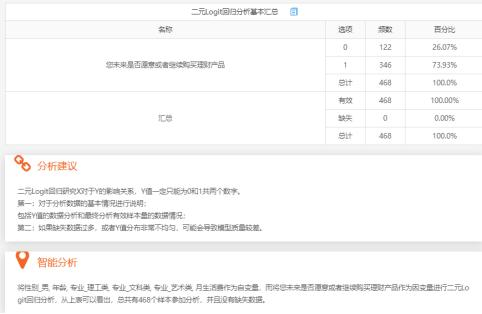二元logit回归分析基本汇总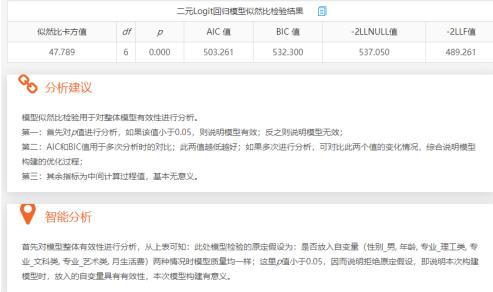二元logit回归模型似然比检验结果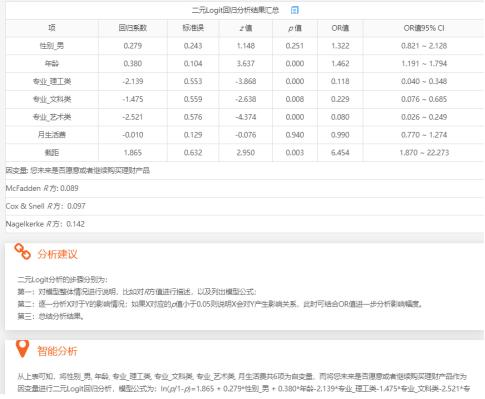二元logit回归结果分析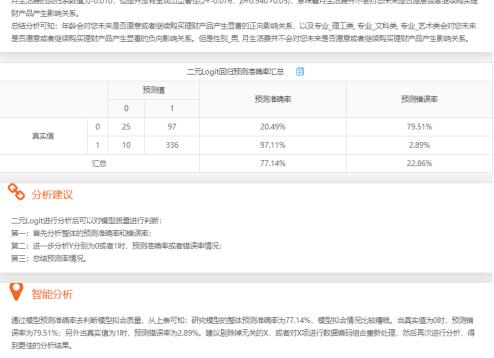二元logit回归预测准确率表格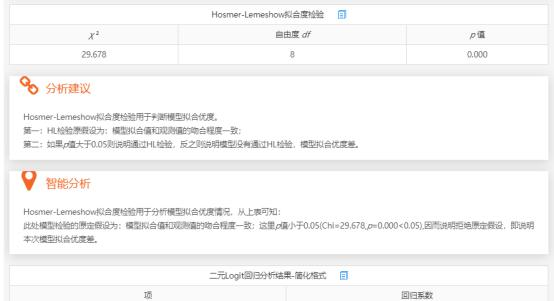Hosmer-Lemeshow拟合度检验
3、二元logistic相关问题
在使用SPSSSAU进行二元logistic回归时，可能会出现一些问题，比如提示奇异矩阵，质量异常，Y值只能为0或1等，接下来一一说明。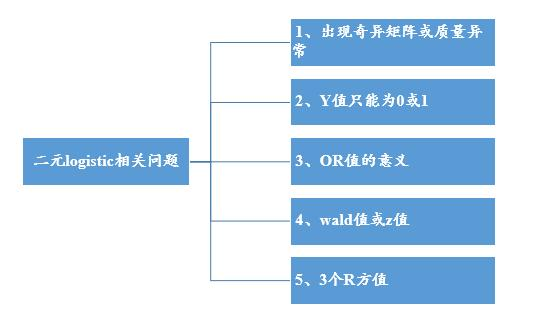第1点：出现奇异矩阵或质量异常
如果做二元logistic提示奇异矩阵，通常有两个原因，一是虚拟哑变量设置后，本应该少放1项作为参考项但是并没有，而是把所有的哑变量项都放入框中，这会导致绝对的共线性问题即会出现奇异矩阵矩阵。二是X之间有着太强的共线性(可使用通用方法的线性回归查看下VIF值)，此时也可能导致模型无法拟合等。先找出原因，然后把有问题的项移出模型中即可。
同时，如果自变量X的分布极其不均匀，SPSSAU建议可先对类别进行组合，可使用数据处理里面的数据编码完成。
第2点：Y值只能为0或1
二元logistic回归研究X对Y的影响，Y为两个类别，比如是否愿意，是否喜欢，是否患病等，数字一定有且仅为2个，分别是0和1。如果不是这样就会出现此类提示，可使用SPSSAU频数分析进行检查，并且使用数据处理->数据编码功能进行处理成0和1。
第3点：OR值的意义
OR值=exp(b)值，即回归系数的指数次方，该值在医学研究里面使用较多，实际意义是X增加1个单位时，Y的增加幅度。如果仅仅是研究影响关系，该值意义较小。
第4点： wald值或z值
z 值=回归系数/标准误，该值为中间过程值无意义，只需要看p 值即可。有的软件会提供wald值(但不提供z 值，该值也无实际意义)，wald值= z 值的平方。
第5点： McFadden R 方、Cox & Snell R 方和Nagelkerke R 方相关问题？
Logit回归时会提供此3个R 方值(分别是McFadden R 方、Cox & Snell R 方和Nagelkerke R 方)，此3个R 方均为伪R 方值，其值越大越好，但其无法非常有效的表达模型的拟合程度，意义相对交小，而且多数情况此3个指标值均会特别小，研究人员不用过分关注于此3个指标值。一般报告其中任意一个R方值指标即可。

展开全文• ## logistic回归模型

万次阅读 多人点赞 2020-04-13 17:44:45
logistic回归模型 从这一期开始，我们准备介绍一系列机器学习算法模型，主要包括logistic回归，决策树，随机森林，关联规则，朴素贝叶斯，支持向量机模型，隐式马尔可夫模型，因子分析，主成分分析，聚类，多元线性...


logistic回归模型
前言logistic回归模型logit变换几率logistic模型二项逻辑回归模型损失函数
logistic回归模型的应用logistic回归模型的评价

前言
从这一期开始，我们准备介绍一系列机器学习算法模型，主要包括logistic回归，决策树，随机森林，关联规则，朴素贝叶斯，支持向量机，隐式马尔可夫，因子分析，主成分分析，聚类，多元线性回归，时间序列，协同过滤，XGBoost，LightGBM，神经网络等，一般会涵盖模型的引入背景，背后数学原理，模型的应用范围，模型优缺点及改进建议，具体工程实践。既适合刚入门机器学习的新手，也适合有一定基础的读者想要进一步掌握模型的核心要义，其中不免会涵盖许多数学符号和公式以及推导过程，如果你觉得晦涩难懂，可以来微信公众号“三行科创”交流群和大家一起讨论。
logistic回归模型
逻辑回归（Logistic Regression）是机器学习中最常见的一种用于二分类的算法模型，由于其数学原理简单易懂，作用高效，其实际应用非常广泛。虽然带回归二字，实则是分类模型，下面从logit变换开始。
logit变换
我们在研究某一结果

y

y

与一系列因素

(

x

1

,

x

2

,

⋯

,

x

n

)

(x_1,x_2,\cdots,x_n)

之间的关系的时候，最直白的想法是建立因变量和自变量的多元线性关系

y

=

θ

0

+

θ

1

x

1

+

θ

2

x

2

+

⋯

+

θ

n

x

n

y=\theta_0+\theta_1x_1+\theta_2x_2+\cdots +\theta_nx_n

其中

(

θ

0

,

θ

1

,

θ

2

,

⋯

,

θ

n

)

(\theta_0,\theta_1,\theta_2,\cdots,\theta_n)

为模型的参数，如果因变量是数值型的话，可以解释成某某因素

x

i

x_i

变化了多少导致结果

y

y

发生了多少变化，如果因变量

y

y

是用来刻画结果是否（0-1）发生？或者更一般的来刻画某特定结果发生的概率（0~1）呢？这时候因素

x

i

x_i

变化导致结果

y

y

的变化恐怕微乎其微，有时候甚至忽略不计。然而实际生活中，我们知道某些关键因素会直接导致某一结果的发生，如亚马逊雨林一只蝴蝶偶尔振动翅膀，就会引起两周后美国德克萨斯州的一场龙卷风。于是，我们需要让不显著的线性关系变得显著，使得模型能够很好解释随因素的变化，结果也会发生较显著的变化，这时候，人们想到了logit变换，下图是对数函数图像从对数函数的图像来看，其在

(

0

,

1

)

(0,1)

之间的因变量的变化是很迅速的，也就是说自变量的微小变化会导致因变量的巨大变化，这就符合了之前想要的效果。于是，对因变量进行对数变换，右边依然保持线性关系，有下面式子

l

o

g

(

y

)

=

θ

0

+

θ

1

x

1

+

θ

2

x

2

+

⋯

+

θ

n

x

n

log(y)=\theta_0+\theta_1x_1+\theta_2x_2+\cdots +\theta_nx_n

虽然上式解决了因变量随自变量变化的敏感性问题，同时也约束了

y

y

的取值范围为

(

0

,

+

∞

)

(0,+\infty)

。我们知道概率是用来描述某件事发生的可能性，一件事情发生与否，更应该是调和对称的，也就是说该事件发生与不发生有对立性，结果可以走向必然发生（概率为1），也可以走向必然不发生（概率为0），概率的取值范围为

(

0

,

1

)

(0,1)

，而等式左边

y

y

的取值范围是

(

0

,

+

∞

)

(0,+\infty)

，所以需要进一步压缩，又引进了几率。
几率
几率（odd)是指事件发生的概率与不发生的概率之比，假设事件 A 发生的概率为

p

p

，不发生的概率为

1

−

p

1-p

，那么事件 A 的几率为

o

d

d

(

A

）

=

p

1

−

p

odd(A）=\frac{p}{1-p}

几率恰好反应了某一事件两个对立面，具有很好的对称性，下面我们再来看一下概率和几率的关系首先，我们看到概率从0.01不断增大到 0.99，几率也从0.01随之不断变大到99，两者具有很好的正相关系，我们再对

p

p

向两端取极限有

lim

⁡

p

→

0

+

(

p

1

−

p

)

=

0

\lim\limits _ {p \to 0^+}(\frac{p}{1-p})=0

lim

⁡

p

→

1

−

(

p

1

−

p

)

=

+

∞

\lim\limits _ {p \to 1^-}(\frac{p}{1-p})=+\infty

于是，几率的取值范围就在

(

0

，

+

∞

)

(0，+\infty)

，这符合我们之前的因变量取值范围的假设。
logistic模型
正因为概率和几率有如此密切对等关系，于是想能不能用几率来代替概率刻画结果发生的可能性大小，这样既能满足结果对特定因素的敏感性，又能满足对称性 ，便有了下面式子

l

o

g

(

p

1

−

p

)

=

θ

0

+

θ

1

x

1

+

θ

2

x

2

+

⋯

+

θ

n

x

n

log(\frac{p}{1-p}) =\theta_0+ \theta_1x_1+\theta_2x_2+\cdots +\theta_nx_n

现在，我们稍微改一改，让等式左边对数变成自然对数

l

n

=

l

o

g

e

ln=log_e

，等式右边改成向量乘积形式，便有

l

n

(

p

1

−

p

)

=

θ

X

ln(\frac{p}{1-p})=\theta X

其中

θ

=

(

1

,

θ

1

,

θ

2

,

⋯

,

θ

n

)

\theta=(1,\theta_1,\theta_2,\cdots,\theta_n)

，

X

=

(

1

,

x

1

,

x

2

,

⋯

,

x

n

)

T

X=(1,x_1,x_2,\cdots,x_n)^T

，我们解得

p

=

e

θ

X

1

+

e

θ

X

p=\frac{e^{\theta X}} {1+ e^{\theta X}}

其中

e

e

是自然常数，保留5位小数是2.71828。这就是我们常见的logistic模型表达式，作出其函数图像如下我们看到logistic函数图像是一条S型曲线，又名sigmoid曲线，以

(

0

,

0.5

)

(0,0.5)

为对称中心，随着自变量

x

x

不断增大，其函数值不断增大接近1，随自变量

x

x

不断减小，其函数值不断降低接近0，函数的取值范围在

(

0

,

1

)

(0,1)

之间，且函数曲线在中心位置变化速度最快，在两端的变化速率较慢。
从上面的操作，我们可以看到逻辑回归模型从最初的线性回归模型基础上对因变量进行 logit 变换，使得因变量对自变量显著，同时约束因变量取值范围为0到正无穷大，然后用概率表示几率，最后求出概率关于自变量的表达式，把线性回归的结果压缩在

(

0

,

1

)

(0,1)

范围内，这样最后计算出的结果是一个0到1之间的概率值，表示某事件发生的可能性大小，可以做概率建模，这也是为什么逻辑回归叫逻辑回归，而不叫逻辑分类了。
二项逻辑回归模型
既然logistic回归把结果压缩到连续的区间

(

0

,

1

)

(0,1)

，而不是离散的 0 或者1，然后我们可以取定一个阈值，通常以0.5为阈值，通过对比概率值与阈值的大小关系而定，如果计算出来的概率大于0.5，则将结果归为一类 （1），如果计算出来的概率小于0.5，则将结果归为另一类 （0），用分段函数写出来便是

y

=

{

1

p > 0.5

0

p < 0.5

y= \begin{cases} 1&\text{p > 0.5} \\ 0 & \text{p < 0.5} \end{cases}

这样逻辑回归就可以用来进行2分类了，在本文中，不特别指出，均指二项逻辑回归，假设数据结构如下
样本

x

1

x_1

x

2

x_2

…

x

n

x_n

y

y

1

x

11

x_{11}

x

12

x_{12}

…

x

1

n

x_{1n}

y

1

y_1

2

x

21

x_{21}

x

22

x_{22}

…

x

2

n

x_{2n}

y

2

y_2

⋮

\vdots

⋮

\vdots

⋮

\vdots

…

⋮

\vdots

⋮

\vdots

m

x

m

1

x_{m1}

x

m

2

x_{m2}

…

x

m

n

x_{mn}

y

m

y_m

其中

m

m

表示样本个数，

n

n

表示影响因数的个数，

y

i

(

i

=

0

,

1

,

⋯

,

m

)

y_i(i=0,1,\cdots,m)

取 0 或者 1。现在我们结合数据，利用条件概率分布模型给出基于概率的二项logistic模型如下

p

(

y

=

1

∣

X

;

θ

)

=

e

θ

X

1

+

e

θ

X

p(y=1|X;\theta)=\frac{e^{\theta X}}{1+e^{\theta X}}

p

(

y

=

0

∣

X

;

θ

)

=

1

1

+

e

θ

X

p(y=0|X;\theta)=\frac{1}{1+e^{\theta X}}

其中，

X

X

表示自变量，

y

y

表示因变量所属的类别，

θ

\theta

为模型待求的参数，模型解释为在特定的因素下，模型结果取1的概率和取0的概率。模型建好了，接下来就需要进行机器训练，而怎么来给训练一种恰当反馈呢？答案是损失函数，通过损失函数来评估模型学习的好坏和改进机制。
损失函数
由前面阈值的取定原则，我们知道相当于我们用一个类别值代替概率值，而类别值是sigmoid 函数的两个最值，概率不可能时时刻刻都取到最值，这势必会造成误差，我们把这种误差称为损失，为了给出损失函数表达式，我们假设模型第

i

i

个样本所求的概率值为

p

i

p_i

，而真实类别值可能是 0 或者 1。
当类别真实值是 1 的情况下，所求的概率值

p

i

p_i

越小，越接近0，被划为类别 0 的可能性越大，被划为类别 1 的可能性越小，导致的损失越大。反之，所求的概率值

p

i

p_i

越大，越接近1，被划为类别 1 的可能性越大，被划为类别 0 的可能性越小，导致的损失越小。我们用下面的函数来描述这种变化关系

−

l

o

g

p

i

-log p_i

其中

p

i

∈

(

0

,

1

)

p_i\in(0,1)

，其图像大致如下当类别真实值是0的情况下，所求的概率值

p

i

p_i

越大，越接近1，其结果的类别判定更偏向于1，导致的损失越大。反之，所求的概率值

p

i

p_i

越小，越接近0，其结果的类别判断更偏向于 0，导致的损失越小。我们用下面的函数来描述这种变化关系

−

l

o

g

(

1

−

p

i

)

-log(1-p_i)现在就要把两种情况结合起来，就不需要分真实值是 1 还是 0 两种情况讨论，求出其期望值，做成一个交叉熵(cross entropy)的整体损失函数如下

c

o

s

t

i

=

−

y

i

l

o

g

(

p

i

)

−

(

1

−

y

i

)

l

o

g

(

1

−

p

i

)

cost_i = -y_ilog(p_i)-(1-y_i)log(1-p_i)

其中

y

i

y_i

表示第

i

i

个样本的真实值，

p

i

p_i

是根据模型计算出来的概率值，当

y

i

=

1

y_i=1

时，

c

o

s

t

i

=

−

l

o

g

(

p

i

)

cost_i= -log(p_i)

，当

y

i

=

0

y_i=0

时，

c

o

s

t

i

=

−

l

o

g

(

1

−

p

i

)

cost_i = -log(1-p_i)

，这符合前面两种情况。
假设现在有

m

m

个样本，总体的损失函数为

C

o

s

t

=

∑

i

=

1

m

c

o

s

t

i

=

∑

i

=

1

m

[

−

y

i

l

o

g

(

p

i

)

−

(

1

−

y

i

)

l

o

g

(

1

−

p

i

)

]

\begin{aligned} Cost &= \sum\limits_{i=1}^m cost_i \\ &=\sum\limits_{i=1}^m[-y_ilog(p_i)-(1-y_i)log(1-p_i)]\\ \end{aligned}

代入

p

i

=

e

θ

X

1

+

e

θ

X

p_i=\frac{e^{\theta X}}{1+e^{\theta X}}

得

C

o

s

t

=

∑

i

=

1

m

[

−

y

i

l

o

g

(

e

θ

X

1

+

e

θ

X

)

−

(

1

−

y

i

)

l

o

g

(

1

−

e

θ

X

1

+

e

θ

X

)

]

\begin{aligned} Cost &=\sum\limits_{i=1}^m[-y_ilog(\frac{e^{\theta X}}{1+e^{\theta X}})-(1-y_i)log(1-\frac{e^{\theta X}}{1+e^{\theta X}})] \end{aligned}

上式就是二项逻辑回归的损失函数，是一个关于参数

θ

\theta

和

X

X

的二元函数，也叫对数似然函数，现在问题转化为以对数似然函数为目标函数的最优化问题，其中

θ

\theta

为模型待求的参数，为了求参数

θ

\theta

，可以对目标函数求偏导数，记

L

(

X

∣

θ

)

=

∑

i

=

1

m

[

−

y

l

o

g

(

e

θ

X

1

+

e

θ

X

)

−

(

1

−

y

)

l

o

g

(

1

−

e

θ

X

1

+

e

θ

X

)

]

\mathcal{L}(X|\theta)=\sum\limits_{i=1}^m[-ylog(\frac{e^{\theta X}}{1+e^{\theta X}})-(1-y)log(1-\frac{e^{\theta X}}{1+e^{\theta X}})]

对

L

(

X

∣

θ

)

\mathcal{L} (X|\theta)

求关于

θ

\theta

的偏导，主要是里面对数函数关于

θ

\theta

的偏导数求解

∂

L

(

X

∣

θ

)

∂

θ

=

∂

{

∑

i

=

1

m

[

−

y

i

l

o

g

(

e

θ

X

1

+

e

θ

X

)

−

(

1

−

y

i

)

l

o

g

(

1

−

e

θ

X

1

+

e

θ

X

)

]

}

∂

θ

=

∑

i

=

1

m

[

(

−

y

i

)

∂

l

o

g

(

e

θ

X

1

+

e

θ

X

)

∂

θ

−

(

1

−

y

i

)

∂

l

o

g

(

1

−

e

θ

X

1

+

e

θ

X

)

∂

θ

]

=

∑

i

=

1

m

[

(

−

y

i

)

c

X

1

+

e

θ

X

−

(

1

−

y

i

)

(

−

c

X

e

θ

X

1

+

e

θ

X

)

]

\begin{aligned} &\frac{\partial \mathcal{L}(X|\theta)}{ \partial \theta} \\&=\frac{ \partial \{\sum\limits_{i=1}^m[-y_ilog(\frac{e^{\theta X}}{1+e^{\theta X}})-(1-y_i)log(1-\frac{e^{\theta X}}{1+e^{\theta X}})]\}}{\partial \theta}\\ &= \sum\limits_{i=1}^m[(-y_i)\frac{\partial log(\frac{e^{\theta X}}{1+e^{\theta X}})}{\partial \theta}-(1-y_i)\frac{\partial log(1-\frac{e^{\theta X}}{1+e^{\theta X}})}{\partial \theta}]\\ &= \sum\limits_{i=1}^m [ (-y_i)\frac{cX}{1+e^{\theta X}}-(1-y_i)(-\frac{cXe^{\theta X}}{1+e^{\theta X}})] \end{aligned}

其中

c

=

l

n

a

c=ln a

，

a

a

为对数底数，令

∂

L

(

(

X

∣

θ

)

∂

θ

=

0

\frac{\partial \mathcal{L}((X|\theta)}{ \partial \theta}=0

，求出

θ

\theta

值，然后带入模型便是学到的logistic模型，假设

θ

^

\hat{\theta}

是上式子所求的值，那么原二项logistic模型方程为

p

(

y

=

1

∣

X

,

θ

^

)

=

e

θ

^

X

1

+

e

θ

^

X

p(y=1|X,\hat{\theta})=\frac{e^{\hat{\theta} X}}{1+e^{\hat{\theta} X}}

p

(

y

=

0

∣

X

,

θ

^

)

=

1

1

+

e

θ

^

X

p(y=0|X,\hat{\theta})=\frac{1}{1+e^{\hat{\theta} X}}

logistic回归模型的应用
既然logistic回归主要用来二分类，那么凡是涉及到二分类的场景都有logistic回归的用武之地，同时，我们还可以用logistic回归模型预测某件事情的发生概率，我们常见的logistic回归模型的应用场景有 (1) 在P2P，汽车金融等领域，根据申请人的提供的资料，预测其违约的可能性大小，进而决定是否给其贷款。 (2) 电商平台根据用于购买记录预测用户下一次是否会购买某件商品。 (3) 天涯，bbs，微博，豆瓣短评等舆论平台做情感分类器。如根据某网友对某些特定主题的历史评论数据，预测其下次对某类型的主题是否会给出正面的评论。 (4) 在医疗领域，根据病人症状，预测其肿瘤是良性的还是恶性的。 (5) 根据CT，流行病学，旅行史，检测试剂结果等特点预测某位疑似病人是否真感染新型冠状病毒。 (6) 在精准营销领域，预测某个产品的收益。
logistic回归模型的评价
从logistic回归的数学原理，在阈值取定的时候一刀切的做法上面，我们能够根据logistic回归的这些特点给出模型优缺点评价
优点：
原理简单，模型清晰，操作高效，背后的概率的推导过程经得住推敲，在研究中，通常以 Logistic 回归模型作为基准，再尝试使用更复杂的算法，可以在大数据场景中使用。使用online learning的方式更新轻松更新参数，不需要重新训练整个模型基于概率建模，输出值落在0到1之间，并且有概率意义求出来的参数

θ

i

\theta_i

代表每个特征对输出的影响，可解释性强解决过拟合的方法很多，如L1、L2正则化，L2正则化就可以解决多重共线性问题
缺点：
对数据依赖性强，很多时候需要做特征工程，且主要用来解决线性可分问题因为它本质上是一个线性的分类器，所以处理不好特征之间相关的情况，对模型中自变量多重共线性较为敏感，例如两个高度相关自变量同时放入模型，可能导致较弱的一个自变量回归符号不符合预期，符号被扭转，正好变负号。logit变换过程是非线性的，在两端随着变化率微乎其微，而中间的变化很大，很敏感。 导致很多区间的变量变化对目标概率的影响没有区分度，很难确定阀值。当特征空间很大时，性能不好容易欠拟合，精度不高
下一期，我们将用一个实例来一睹logistic魅力。
参考文献
1，对数函数 2，python绘制对数函数 3，如何理解logistic函数 4，logit究竟是个啥？ 5，逻辑回归 6，从原理到代码，轻松深入逻辑回归 7，https://www.zybuluo.com/frank-shaw/note/143260展开全文损失函数 适用范围
• ## Logistic回归模型

千次阅读 2020-04-03 12:06:39
为什么会用到logistic回归模型 普通线性回归模型的一个假设条件是当自...logistic回归模型可分为二分类和分类模型。 二分类logistic回归模型 当因变量只有0，1两种取值时，因变量服从伯努利分布： 预测自变量取...
为什么会用到logistic回归模型
普通线性回归模型的一个假设条件是当自变量取值确定时，因变量服从方差不变的正态分布。而如果因变量是类别变量，则该假设条件无法满足。为了通过自变量的线性组合来预测类别因变量的取值，logistic回归模型应运而生。logistic回归模型可分为二分类和多分类模型。
二分类logistic回归模型
当因变量只有0，1两种取值时，因变量服从伯努利分布：预测自变量取固定值时y的取值，实际上就是要预测自变量取固定值时y所服从的伯努利分布的p值，即y=1的概率值。因为p的取值范围为[0,1]，所以p作为因变量的函数值域应为[0,1].logistic函数符合这一要求。
logistic函数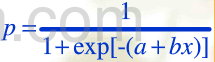函数性质：
函数取值在（0，1）内，上限p=1，下限p=0是它的两条水平渐进馅b>0时单调递增，b<0时单调递减x=-a/b时，p=0.5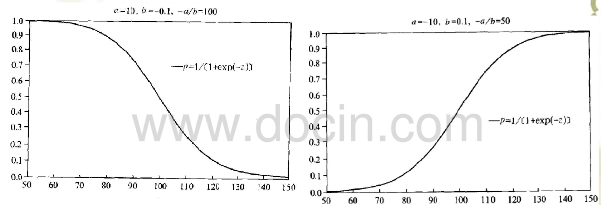因此利用logistic函数构建用于预测p的回归模型。
logistic回归模型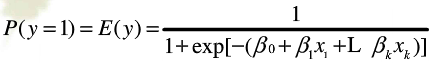其他形式：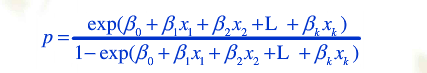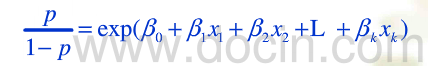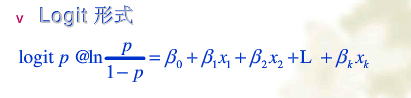logistic回归系数的意义
1.发生比(odds)2. 发生比率（odds ratio OR 或称相对风险比）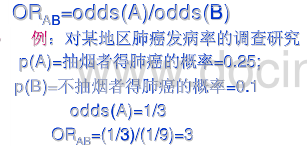3. 变量回归系数的意义
当其他的解释变量保持不变时，解释变量x2每变化一个单位，即x2+1时，原来的y=1的发生比扩大exp（β2）。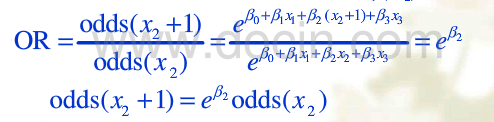当存在交互效应时：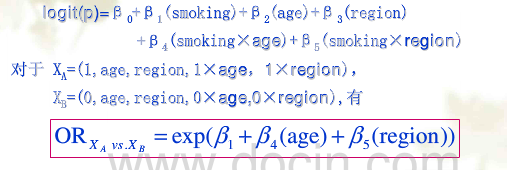logistic回归模型的参数估计与统计检验
参数估计（极大似然估计法）
似然函数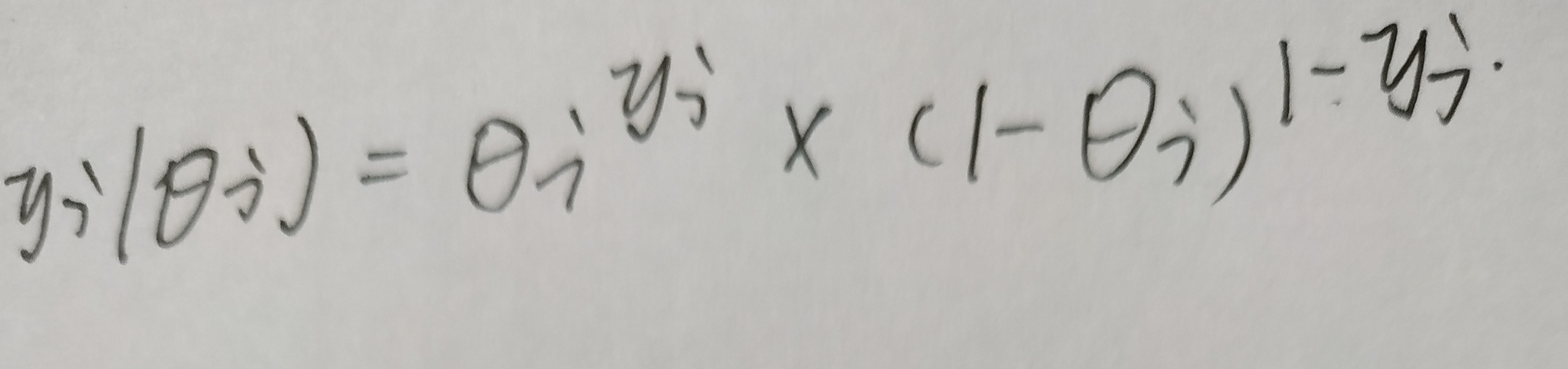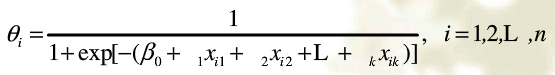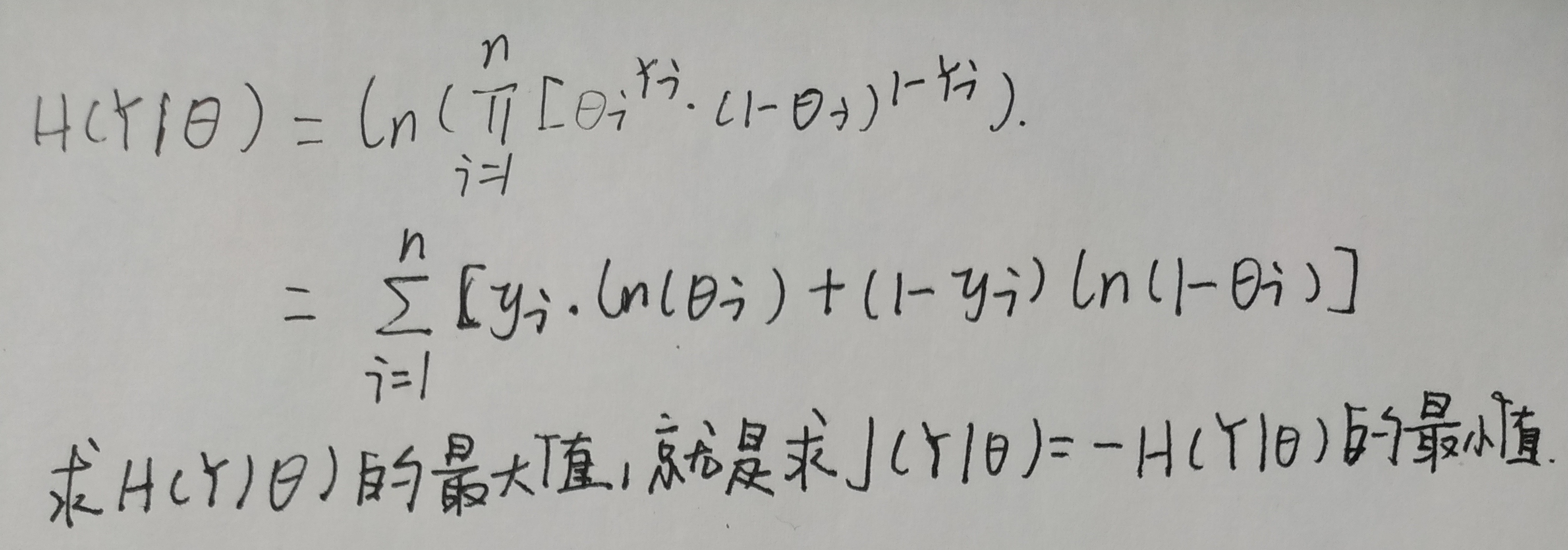梯度下降法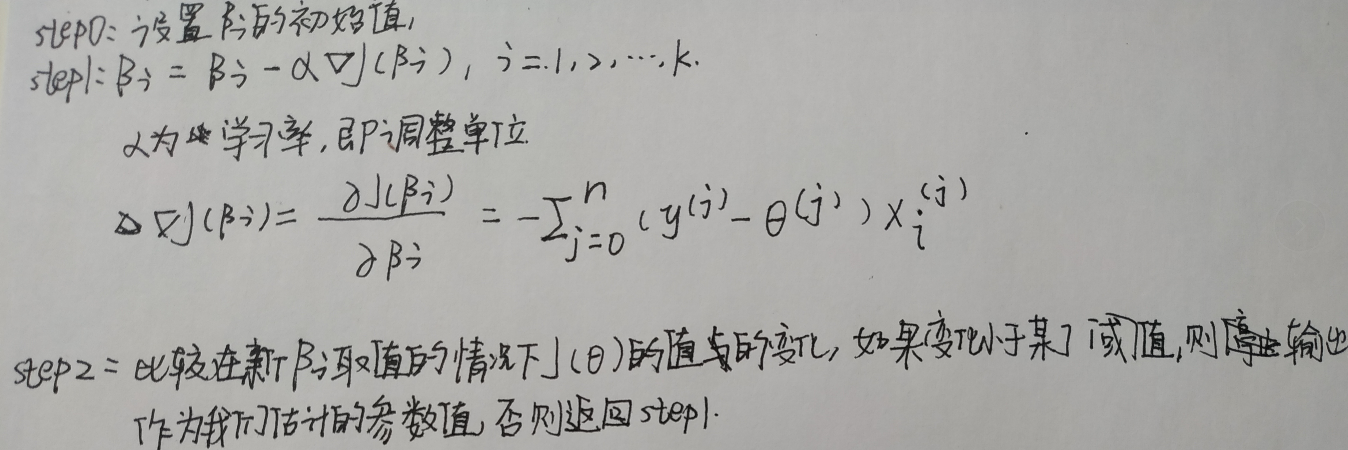统计检验
整体显著性检验
（1）-2 Log Likehood（-2LL）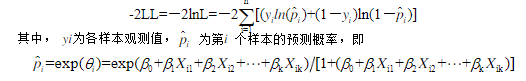-2LL越小，说明模型拟合得越好 （2）似然比L0/L的卡方检验 L0是解释变量均未引入回归方程前的似然函数值 L是解释变量引入回归方程后的似然函数值 H0：L0/L=1 -ln(L0/L)^2近似服从卡方分布
回归系数的显著性检验
（1）wald统计量检测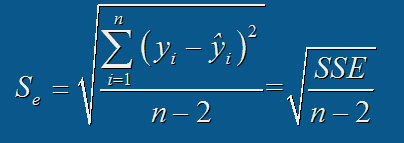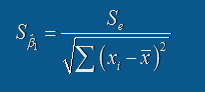H0：βi=0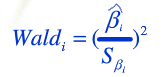wald检验统计量服从自由度为1的卡方分布 wald统计量的缺点：a. 受自变量间多重共线性的影响 b. 如果回归系数绝对值较大，标准误差也会扩大，导致wald检验统计量观测值偏小，不易拒绝零假设 （2）另一种方法 当回归系数绝对值较大时，可以对比较包含该变量和不包含该变量的模型的似然比变化，通过似然比卡方检验来检验该变量的回归系数不为零是否显著。
模型预测能力评价
混淆矩阵
PositiveNegativePositiveTPFNNegativeFPTN
简单评价指标
敏感性（sensitivity）召回率(recall)=TP/(TP+FN) 精确率（precision）=TP/（TP+FP） 准确率（accuracy）=（TP+TN）/（P+N） 特异度（specificity）=TN/(TN+FP)
ROC 曲线
真阳性率=TP/(TP+FN) 假阳性率=FP/(FP+TN) 以假阳性率为横轴，以真阳性率为纵轴，真阳性率和假阳性率会随着正例概率判定阈值的变化而变化，那么不同的阈值就会产生不同的真阳性率和假阳性率，即对应着坐标系中不同的点，将这些点连接起来就绘制好了ROC曲线。 ROC曲线下覆盖的面积越大，则模型的预测能力越高，因为面积越大说明在低阳性率的情况下真阳性率就可以达到较高水平，进一步说明正例的预测发生概率总体相对大于负例的预测发生概率。
正则化
目的：解决过拟合问题 代价函数J加入正则项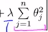放置模型参数过大
多分类Logistic回归模型
选择其中一个类作为参照类，建立k-1个双分类Logistic回归模型表示出其他各类的发生概论和参照类发生概率的比值，进而计算出各类实际发生概率，然后通过极大似然估计法估计各参数值。
展开全文机器学习 分类算法
• 一、 概述 Logistic回归主要用于因变量为分类变量（如疾病的缓解、不缓解，...因变量为二分类的称为二项logistic回归，因变量为分类的称为多元logistic回归。  下面学习一下Odds、OR、RR的概念： 在病例
• Logistic回归是一种统计方法，它可根据输入字段的值对记录进行分类。这种技术与线性回归类似，但用分类目标字段代替了数值字段。 实验目的：例如，假设某个电信提供商根据服务使用情况模式对其客户群进行了细分，将...
• 在这里讲述如何通过MATLAB的代码实现多元logistic回归模型，对于想用MATLAB来实现多元logistic回归模型的朋友有很大的帮助。
• 上一篇文章介绍了线性判别模型，本文介绍线性生成模型——logistic回归模型。本文介绍logstic回归模型相关的知识，为了更好理解模型的决策边界函数，本文同时分析了多元变量的协方差对概率分布的影响。 目录 1、...
• 在医学研究中，为了控制一些重要的混杂因素，经常会把病例和对照按年龄，性别等条件进行配对，...病历对照研究或者倾向得分匹配研究(一种将研究数据处理成‘随机对照实验数据’的方法)中常使用条件Logistic回归进...
• 什么是logistic回归2.损失函数的定义：3.采用梯度下降法求解损失函数4.logistic回归在sklearn中的实现：学习时间：学习产出：一级目录二级目录三级目录 1. 什么是logistic回归 逻辑回归（Logistic Regression），...python 机器学习
• 目录一元线性回归、多元线性回归、Logistic回归、广义线性回归、非线性回归的关系什么是极大似然估计逻辑斯谛回归(Logistic回归)类分类Logistic回归Python代码(sklearn库)一元线性回归、多元线性回归、逻辑斯谛...
• 1. 二分类logistic回归分析 ...无序的类别因变量：对应无序分类logistic回归模型 有序的类别因变量：有序分类logistic回归模型 2.1 无序分类logistic回归分析 2.2 有序分类logistic回归分析 ...
• 在研究Y与X之间的因果关系时，如果Y不是一个定比或定距变量时，就需要进行logistic回归logistic回归是一种广义线性... logistic回归分析类型 因变量Y的特征 举例 二元logistic...
• Logistic回归分析（logit回归）一般可分为3类，分别是二元logistic回归分析分类Logistic回归分析和有序Logistic回归分析logistic回归分析类型如下所示。 Logistic回归分析用于研究X对Y的影响，并且对X...
• R语言无序多分类Logistic回归模型实战 目录 R语言无序多分类Logistic回归模型实战 #导入包 #加载数据数据编码 #模型构建、假设检验、预测推理 #mlogit包多项logit的模型拟合函数 #导入包 ...r语言 机器学习 人工智能 数据挖掘 自然语言处理
• Logistic回归分析（logit回归）一般可分为3类，分别是二元Logistic回归分析分类Logistic回归分析和有序Logistic回归分析logistic回归分析类型如下所示。 Logistic回归分析用于研究X对Y的影响，并且对X的...
• ## logistic回归

千次阅读 2018-10-28 19:04:43
值得一提的是，logistic回归跟多元线性回归有很相似之处，模型形式也都是wx+b，w、b为要求的参数，不同点在于因变量不同，logistic回归通过logistic函数L将wx+b对应一个隐形状态p，即p=L（wx+b），若L是logistic...
• logistic回归模型中提出和讨论了许多评估拟合优度的方法，但是，拟合优度统计量的渐近分布研究较少，需要进行更的研究。 这工作将专注于评估拟合优度检验的渐近分布行为，还将进行全局拟合优度检验之间的比较...
• 根据因变量得离散特征：常用得Logistic回归分析方法有3终，包括二元Logistic回归分析、多元Logistic回归分析以及有序Logistic回归分析等。 13.1二元Logistic回归分析 我们经常会遇到因变量只有两种取值的情况，...statistics
• 我们知道可以通过回归模型进行拟合，并挖掘各个特征变量对模板变量的影响机制，但是，对于分类问题，由于目标变量是离散型变量，因此，不能直接应用回归模型进行解决，这时，可以使用Logistic回归模型进行解决。...
• logistic回归模型是最成熟也是应用最广泛的分类模型，通过学习和实践拟通过从入门、进阶到高级的过程对其进行总结，以便加深自己的理解也为对此有兴趣者提供学习的便利。 一、有关logistic的基本概念 　...
• 因变量为无序分类...无序分类的logistic回归模型与有序分类的logistic回归模型不同。有序分类的logistic回归采用的是累积logit模型，进行logit变换的是因变量有序取值水平的累积概率；而无序分类的logisti
• ## logistic回归模型

千次阅读 2012-09-04 21:23:52
logistic regression是统计学习中...回归模型： 给定一个数据集合(x1,y1)(x2,y2)...(xn,yn)，有监督学习构建模型，学习过程就是模型参数θ的学习过程。作为discrimination algorithm，对 P(Y|X;θ)建模，分布式应用 dataset algorithm input 算法
• 3 Softmax回归模型参数化的特点 4 权重衰减 5 Softmax回归与Logistic 回归的关系 6 Softmax 回归 vs. k 个二元分类器 7 中英文对照 8 中文译者 转自：http://ufldl.stanford.edu/...
• Logistic回归：实际上属于判别分析，因拥有很差的判别效率而不常用。 1． 应用范围： ① 适用于流行病学资料的危险因素分析 ② 实验室中药物的剂量-反应关系 ③ 临床试验评价 ④ 疾病的预后因素分析 2． ...
• ## 用R语言建立logistic回归模型

万次阅读 多人点赞 2019-02-20 22:13:32...